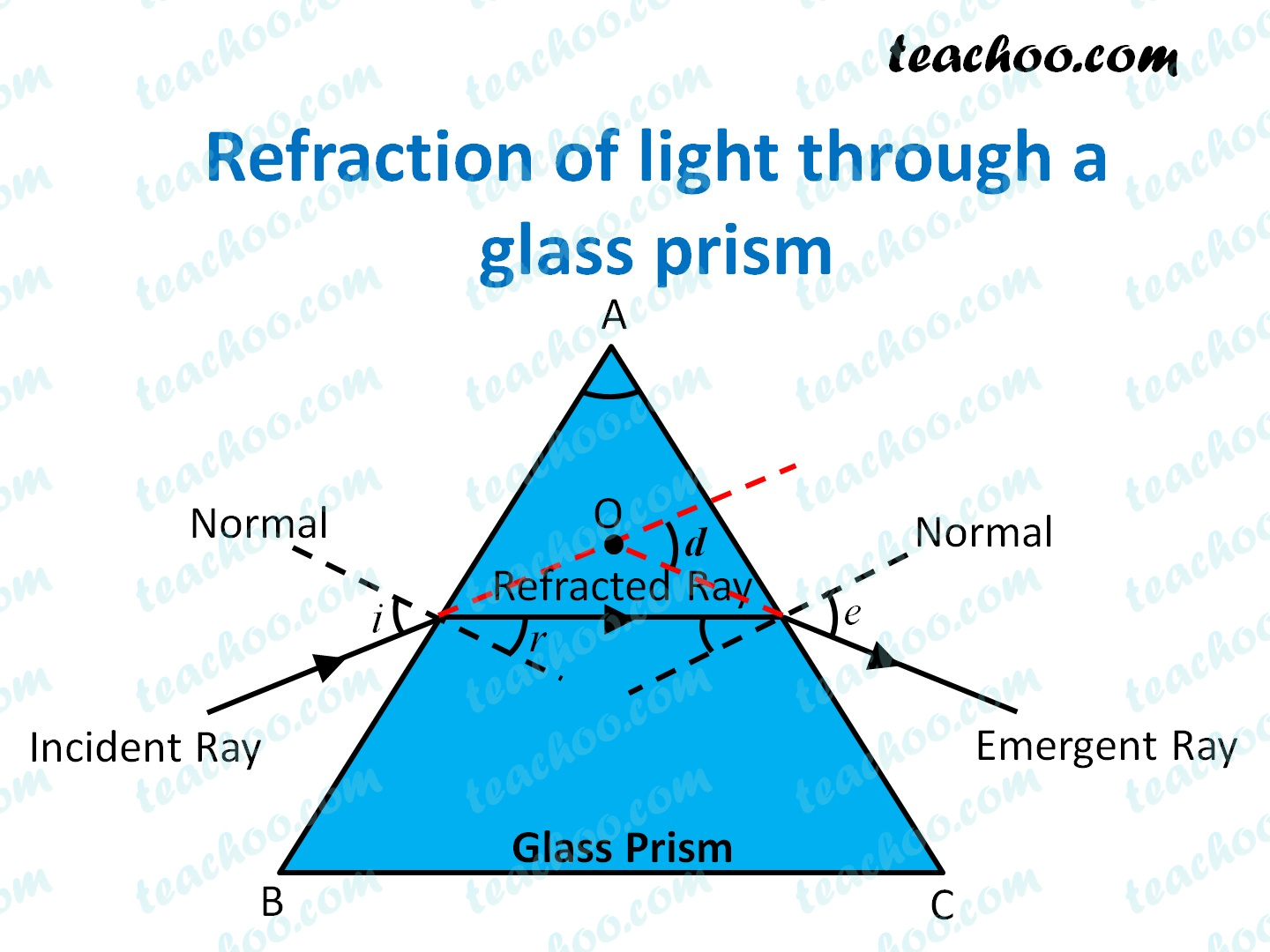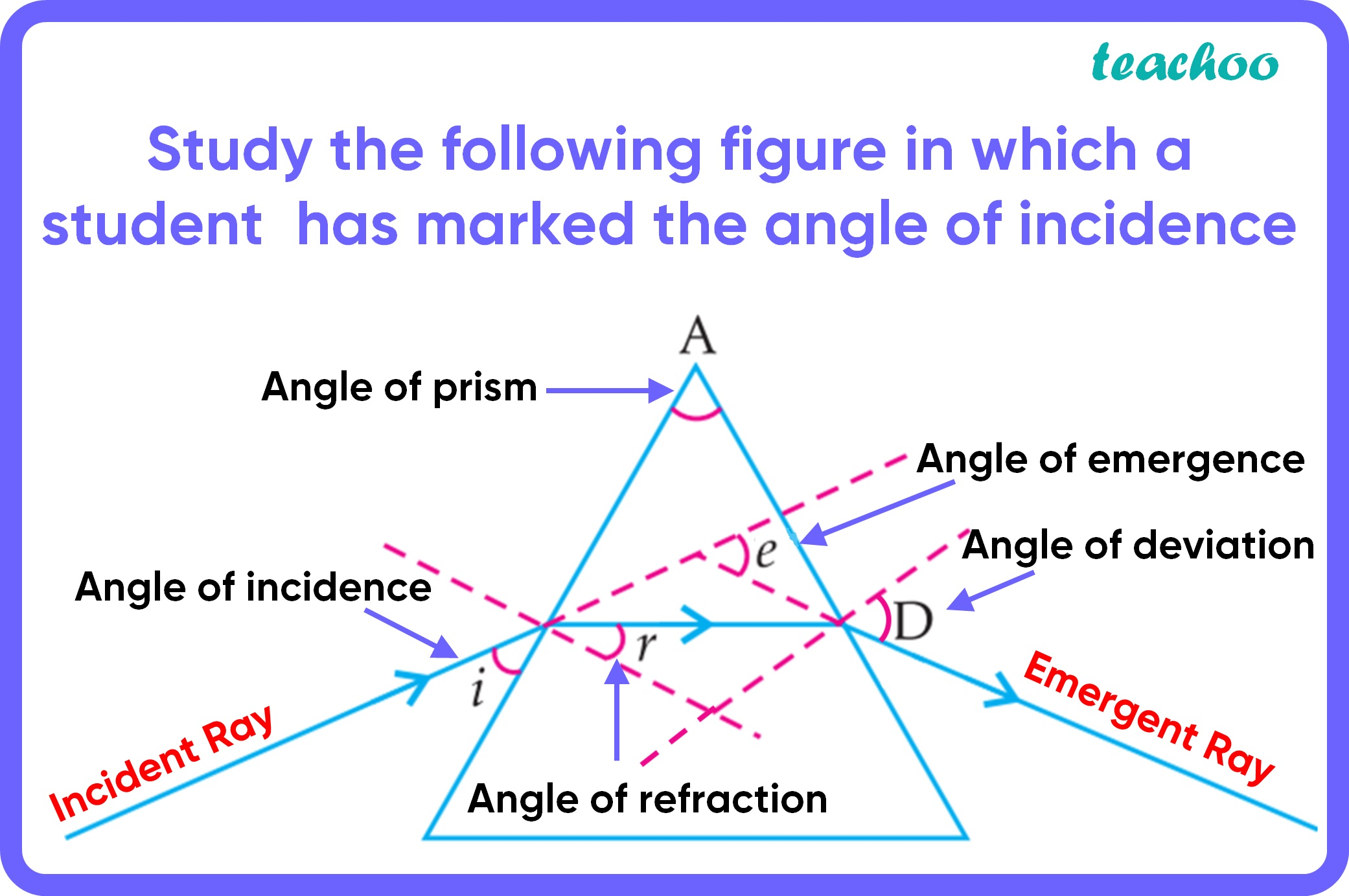MCQ from Past Year Papers

Class 10
Chapter 10 Class 10 - Light - Reflection and Refraction

## (d) ∠A, ∠i, ∠r and ∠D• Angle of incidence is the angle between incident ray and normal.
So, here angle of incidence is angle i. It is marked correct

• Angle of refraction is the angle between refracted ray and normal.
So, here angle of incidence is angle r. It is marked incorrectly

• Angle of emergence is the angle between emergent ray and normal.
So, here angle of emergence is angle D, not angle e. So, it is marked incorrectly

• Angle of deviation is the angle between emergent ray and incident ray (when produced backwards).
So, here angle of emergence is angle e, not angle D. So, it is marked incorrectly

• Angle of prism is the vertex angle of the prism.
Here, it is angle A. It is marked correctSo, the correct answer is (a) - ∠A and ∠i

Learn in your speed, with individual attention - Teachoo Maths 1-on-1 Class